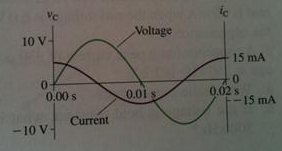# Problem: The figure shows voltage and current graphs for a capacitor. (a) What is the emf frequency f? (b) What is the value of the capacitance C? (c) Draw the capacitor's voltage and current phasors at t = 7.5 ms.

###### FREE Expert Solution

(a) Frequency:

$\overline{){\mathbf{f}}{\mathbf{=}}\frac{\mathbf{1}}{\mathbf{T}}}$

T = 0.02s

94% (273 ratings)###### Problem Details

The figure shows voltage and current graphs for a capacitor.

(a) What is the emf frequency f?

(b) What is the value of the capacitance C?

(c) Draw the capacitor's voltage and current phasors at t = 7.5 ms.